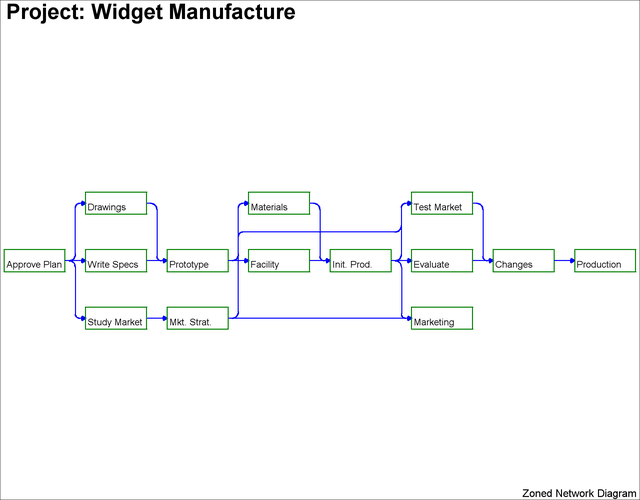Example 9.2 Graphics Version of PROC NETDRAW

The same network used in Example 9.1 is drawn here with the GRAPHICS option (which is also the default mode of output for the NETDRAW procedure). In this example, the network is drawn before scheduling the project with PROC CPM. The global options HPOS= and VPOS= are set to 100 and 70, respectively. These options control the number of character cell positions on the screen. The network is displayed in Output 9.2.1. Note that all the nodes are the same color as specified by the PATTERN statement, the color of the arcs is blue as specified by the CARCS= option, the width of all lines is 3 as specified by the LWIDTH= option.

```goptions hpos=100 vpos=70 border;
pattern1 c=green v=e;
title h=3  j=l ' Project: Widget Manufacture';
proc netdraw data=widget graphics;
actnet / act=task succ=(succ1 succ2 succ3)
height=1.5 compress
carcs=blue lwidth=3;
run;
```

Output 9.2.1: Project Network